Next: Pulse Propagation Up: Waves in Cold Plasmas Previous: Resonant Layers

# Collisional Damping

Let us now consider a real-life damping mechanism. Equation (464) specifies the linearized Ohm's law in the collisionless cold-plasma approximation. However, in the presence of collisions this expression acquires an extra term (see Sect. 3), such that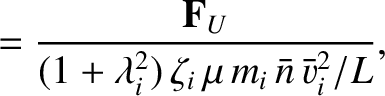(614)

where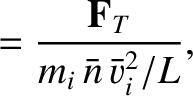is the collision frequency. Here, we have neglected the small difference between the parallel and perpendicular plasma electrical conductivities, for the sake of simplicity. When Eq. (614) is used to calculate the dielectric permittivity for a right-handed wave, in the limit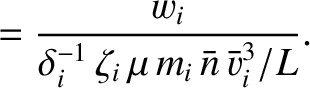, we obtain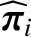(615)

A right-handed circularly polarized wave, propagating parallel to the magnetic field, is governed by the dispersion relation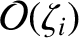(616)

Suppose that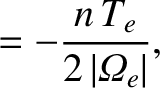. Furthermore, let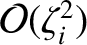(617)

so that the electron cyclotron resonance is located at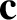. We also assume that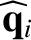, so that the evanescent region corresponds to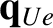. It follows that in the immediate vicinity of the resonance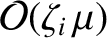(618)

where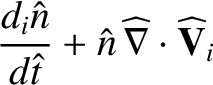(619)

and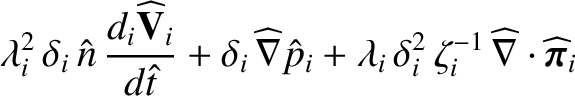(620)

It can be seen that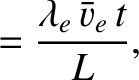, which is consistent with the absorption of incident wave energy by the resonant layer. The approximate width of the resonant layer is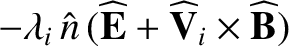(621)

Note that the damping mechanism, in this case collisions, controls the thickness of the resonant layer, but does not control the amount of wave energy absorbed by the layer. In fact, in the simple theory outlined above, all of the incident wave energy is absorbed by the layer.Next: Pulse Propagation Up: Waves in Cold Plasmas Previous: Resonant Layers
Richard Fitzpatrick 2011-03-31